# Bihar Board Class 9th Maths Solutions Chapter 5 Introduction to Euclid’s Geometry Ex 5.1

Bihar Board Class 9th Maths Solutions Chapter 5 Introduction to Euclid’s Geometry Ex 5.1 Textbook Questions and Answers.

## BSEB Bihar Board Class 9th Maths Solutions Chapter 5 Introduction to Euclid’s Geometry Ex 5.1Question 1.
Which of the following statements are true and which are false? Give reasons for your answers?
(i) Only one line can pass through a single point.
(ii) There are an infinite number of lines which pass through two distinct points.
(iii) A terminated line can be produced indefinitely on both the sides,
(iv) If two circles are equal, then their radii are equal.
(v) In Figure, if AB = PQ and PQ = XY, then AB = XY.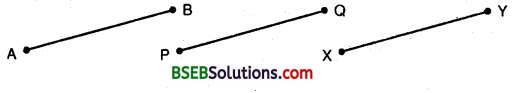Solution:
(i) False.
Mark a point P on the plane of paper. Using a sharp pencil and a ruler, draw a line l passing through it as shown in the figure. Draw another line m passing through P. Continuing this process, we can draw as many lines as we please, each passing through point P. Thus, an infinite number of lines can be drawn passing through a given point.(ii) False.
Mark two points A and B on the plane of paper. Fold the paper so that a crease passes through A. As explained in part (i) an unlimited number of creases (lines) can pass through A.Again, fold the paper so that a crease passes through B. Clearly, an unlimited number of creases (lines) can pass through B. Now, fold the paper in such a way that a crease (line) passes through both A and B. We observe that there is just one crease (line) which passes through both A and B.
Thus, through any two points in a plane, exactly one line can be drawn.

(iii) True.
Note that what we call a line segment now a days is what Euclid’s called a terminated line.In geometry, by a line, we mean the line in its totality and not a portion of it. A physical example of a perfect line is not possible.
Since a line extends indefinitely in both the directions.
So, it cannot be drawn or shown wholly on paper. In practice, only a portion of a line is drawn and arrowheads are marked at its two ends indicating that it extends indefinitely in both directions as shown.

(iv) True.
On supperimposing the region bounded by one circle on the other circle if the circles coincide. Then, their centres and boundaries coincide. Therefore, their radii will be equal.

(v) True.
Because things which are equal to the same thing are equal to one another.Question 2.
Give a definition for each of the following terms. Are there other terms that need to be defined first? What are they, and how might you define them?
(i) parallel lines
(ii) perpendicular lines
(iii) line segment
(v) square
Solution:
For the desired definition we need the following terms:
(a) point
(b) line
(c) plane
(d) ray
(e) angle
(f) circle
It is not possible to define first three precisely. However, a good idea of these concepts shall be given.
(a) A small dot made by a sharp pencil on a sheet paper gives an idea about a point. A point has no dimension, it has only a position.

(b) A straight crease obtained by folding a paper, a straight string pulled at its two ends, the edge of a ruler are some close examples of a geometrical line. The basic concept about a line is that it should be straight and that it should extend in definitely in both the directions.

(c) The surface of a smooth wall or the surface of a sheet of paper are close examples of a plane.

(d) A part of line l which has only one end-point A and contains the point B is called a ray AB.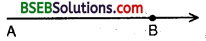(e) An angle is the union of two non-collinear rays with a common initial point.

(f) A. circle is the set of all those points in a plane whose distance from a fixed point remains constant. The fixed point is called the centre of the circle.

(g) A closed figure made of four line segments is called a quadrilateral.
(i) Parallel Lines : Two lines are said to be parallel when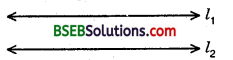(a) they are intersecting
(b) they are caplanar.
In figure, the two lines l1 and l2 are parallel.

(ii) Perpendicular Lines : Two lines AB and CD lying the same plane are said to be perpendicular, if they form a right angle. We write AB ⊥ CD.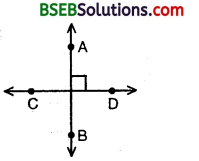(iii) Line segment: A line segment is a part of line. When two distinct points, say A and B on a line are given, then the part of this line with end-points A and B is called the line segment. It is named as $$\overline{A B}$$ and BA denote the same line segment.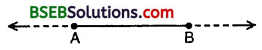(iv) Radius : The distance from .the centre to a point on the circle is called the radius of the circle. OP is the radius.(v) Square : A quadrilateral in which all the four angles are right angles and four sides are equal is called a square. ABCD is a square of a circle.Question 3.
Consider two ‘postulates’ given below :
(i) Given any two distinct points A and B, there exists a third point C which is in between A and B.
(ii) There exist at least three points that are not on the same line.
Do these postulates contain any undefined terms? Are these postulates consistent?
Do they follow from Euclid’s postulates? Explain.
Solution:
There are several undefined terms which the student should list. They are consistent, because they deal with two different situations :

• says that the given two points A and B, there is a point C lying on the line in between them;
• says that given A and B, we can take C not lying on the line through A and B.

These ‘postulates’ do not follow from Euclid’s postulates. However, they follow from axiom stated as given two distinct points, there is a unique line that passes through them.

Question 4.
If a point C lies between two points A and B such that AC = BC, then prove that AC = $$\frac { 1 }{ 2 }$$ AB. Explain by drawing the figure.
Solution:
We have-a point C lying between two points Aj and B such, that AC = BC.
Adding AC on both sides, we have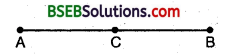AC + AC = AC + BC
⇒ 2 AC = AB [∵ AC + CB coincides withAB]
AC = $$\frac { 1 }{ 2 }$$ AB.

Question 5.
In Question 4, point C is called a mid-point of line segment AB. Prove that every line segment has one and only one mid-point.
Solution:
If possible, let D be another mid-point of AB.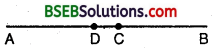But it is given that C is the mid-point of AB.
∴ AC = CB …(2)
Subtracting (1) from (2), we get
AC – AD = CB – DB
⇒ DC = – DC
⇒ 2DC = 0 ⇒ DC = 0
∴ C and D coincides.
Thus, every line segment has one and only one mid-point.Question 6.
In Figure, if AC BD, then prove that AB = CD.Solution:
AC = BD … (1) [Given]
Also AC = AB + BC … (2)
[Point B lies between A and C]
and, BD = BC + CD … (3)
[Point C lies between B and D]
Substituting for AC and BD from (2) and (3) in (1), we
AB + BC = BC + CD
⇒ AB + BC – BC = BC + CD – BC
⇒ AB = CD.Question 7.
Why is Axiom 5, in the list of Euclid’s axioms, considered a ‘universal truth’? (Note that the question is not about the 5th postulate.)
Solution:
Axiom 5 in the list of Euclid’s axioms, is true for any thing in any part of universe so this is a universal truth.